Sections
Test Prep

# Test Prep

### Multiple Choice

#### 19.1Ohm's Law

1.
What are the SI units for electric current?
1. $C/s$
2. $e/s$
3. $−e/s$
4. $C/s2$
2.
What is the SI unit for resistance?
1. $C/m$
2. $C/s$
3. $Ω$
4. $Ψ$
3.

The equivalent unit for an ohm is a ________.

1. V/A
2. C/m
3. $AVAV$
4. V/s
4.
You put $9.0V$ DC across resistor $R1$ and measure the current through it. With the same voltage across resistor $R2$, you measure twice the current. What is the ratio $R1R2$?
1. 1
2. $12$
3. 4
4. 2

#### 19.2Series Circuits

5. What does the circuit element shown represent?
Figure 19.30

1. a battery
2. a capacitor
3. the ground
4. a switch
6.

How many 10-Ω resistors must be connected in series to make an equivalent resistance of 80 Ω?

1. 80
2. 8
3. 20
4. 40
7. Which two circuit elements are represented in the circuit diagram?
Figure 19.31
1. a battery connected in series with an inductor
2. a capacitor connected in series with a resistor
3. a resistor connected in series with a battery
4. an inductor connected in series with a resistor
8.

How much current will flow through a 10-V battery with a 100-Ω resistor connected across its terminals?

1. 0.1 A
2. 1.0 A
3. 0
4. 1,000 A

#### 19.3Parallel Circuits

9.

A 10-Ω resistor is connected in parallel to another resistor R . The equivalent resistance of the pair is 8 Ω. What is the resistance R?

1. 10 Ω
2. 20 Ω
3. 30 Ω
4. 40 Ω
10. Are the resistors shown connected in parallel or in series? Explain.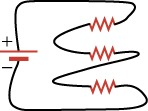1. The resistors are connected in parallel because the same current flows through all three resistors.
2. The resistors are connected in parallel because different current flows through all three resistors.
3. The resistors are connected in series because the same current flows through all three resistors.
4. The resistors are connected in series because different current flows through all three resistors.

#### 19.4Electric Power

11.
Which equation below for electric power is incorrect?
1. $P=I2R$
2. $P=VR2$
3. $P=IV$
4. $P=V2R$
12.
What power is dissipated in a circuit through which $0.12A$ flows across a potential drop of $3.0V$?
1. $0.36W$
2. $0.011W$
3. $5V$
4. $2.5W$
13.
How does a resistor dissipate power?
1. A resistor dissipates power in the form of heat.
2. A resistor dissipates power in the form of sound.
3. A resistor dissipates power in the form of light.
4. A resistor dissipates power in the form of charge.

#### 19.1Ohm's Law

14.

True or false—it is possible to produce nonzero DC current by adding together AC currents.

1. false
2. true
15.

What type of current is used in cars?

1. alternating current
2. indirect current
3. direct current
4. straight current
16.
If current were represented by $C$, voltage by $B$, and resistance by $g$, what would the mathematical expression be for Ohm’s law?
1. $C=Bg$
2. $g=BC$
3. $BC=Cg$
4. $B=Cg$
17.
Give a verbal expression for Ohm’s law.
1. Ohm’s law says that the current through a resistor equals the voltage across the resistor multiplied by the resistance of the resistor.
2. Ohm’s law says that the voltage across a resistor equals the current through the resistor multiplied by the resistance of the resistor.
3. Ohm’s law says that the resistance of the resistor equals the current through the resistor multiplied by the voltage across a resistor.
4. Ohm’s law says that the voltage across a resistor equals the square of the current through the resistor multiplied by the resistance of the resistor.
18.

What is the current through a 100-Ω resistor with 12 V across it?

1. 0
2. 0.12 A
3. 8.33 A
4. 1,200 A
19.
What resistance is required to produce $0.15A$ from a $9.0V$ battery?
1. $0.017$
2. 1
3. 60
4. 120

#### 19.2Series Circuits

20.

Given a circuit with one 9-V battery and with its negative terminal connected to ground. The two paths are connected to ground from the positive terminal: the right path with a 20-Ω and a 100-Ω resistor and the left path with a 50-Ω resistor. How much current will flow in the right branch?

1. $91209120$
2. $91009100$
3. $950950$
4. $920920$
21. Through which branch in the circuit shown does the most current flow?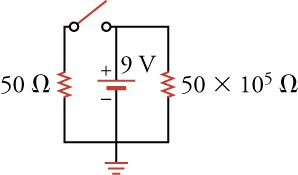1. All of the current flows through the left branch due to the open switch.
2. All of the current flows through the right branch due to the open switch in the left branch.
3. All of the current flows through the middle branch due to the open switch in the left branch
4. There will be no current in any branch of the circuit due to the open switch.
22. What current flows through the $75-Ω$ resistor in the circuit below?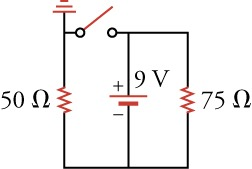1. $0.072A$
2. $0.12A$
3. $0.18A$
4. $0.3A$
23. What is the equivalent resistance for the circuit below if $V=9.0V$ and $I=0.25A$?/span>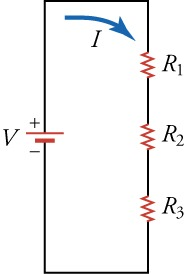1. $0.028Ω$
2. $2.25Ω$
3. $36Ω$
4. $72Ω$

#### 19.3Parallel Circuits

24.

Ten 100-Ω resistors are connected in series. How can you increase the total resistance of the circuit by about 40 percent?

1. Adding two 10-Ω resistors increases the total resistance of the circuit by about 40 percent.
2. Removing two 10-Ω resistors increases the total resistance of the circuit by about 40 percent.
3. Adding four 10-Ω resistors increases the total resistance of the circuit by about 40 percent.
4. Removing four 10-Ω resistors increases the total resistance of the circuit by about 40 percent.
25.
Two identical resistors are connected in parallel across the terminals of a battery. If you increase the resistance of one of the resistors, what happens to the current through and the voltage across the other resistor?
1. The current and the voltage remain the same.
2. The current decreases and the voltage remains the same.
3. The current and the voltage increases.
4. The current increases and the voltage remains the same.
26. In the circuit shown through which resistor(s) does the most current flow? Through which does the least flow? Explain.
Figure 19.32
1. The most current flows through the 15-Ω resistor because all the current must pass through this resistor.
2. The most current flows through the 20-Ω resistor because all the current must pass through this resistor.
3. The most current flows through the 25-Ω resistor because it is the highest resistance.
4. The same current flows through the all the resistor because all the current must pass through each of the resistors.

#### 19.4Electric Power

27.

You want to increase the power dissipated in a circuit. You have the choice between doubling the current or doubling the resistance, with the voltage remaining constant. Which one would you choose?

1. doubling the resistance
2. doubling the current
28.

You want to increase the power dissipated in a circuit. You have the choice between reducing the voltage or reducing the resistance, with the current remaining constant. Which one would you choose?

1. reduce the voltage to increase the power
2. reduce the resistance to increase the power
29.

What power is dissipated in the circuit consisting of 310-Ω resistors connected in series across a 9.0-V battery?

1. The power dissipated is 2430 W.
2. The power dissipated is 270 W.
3. The power dissipated is 2.7 W.
4. The power dissipated is 0.37 W.
30.

What power is dissipated in a circuit consisting of three 10-Ω resistors connected in parallel across a 9.0-V battery?

1. The power dissipated is 270 W.
2. The power dissipated is 30 W.
3. The power dissipated is 24 W.
4. The power dissipated is 1/24 W.

### Extended Response

#### 19.1Ohm's law

31.
Describe the relationship between current and charge. Include an explanation of how the direction of the current is defined.
1. Electric current is the charge that passes through a conductor per unit time. The direction of the current is defined to be the direction in which positive charge would flow.
2. Electric current is the charges that move in a conductor. The direction of the current is defined to be the direction in which positive charge would flow.
3. Electric current is the charge that passes through a conductor per unit time. The direction of the current is defined to be the direction in which negative charge would flow.
4. Electric current is the charges that move in a conductor. The direction of the current is defined to be the direction in which negative charge would flow.
32.
What could cause Ohm’s law to break down?
1. If small amount of current flows through a resistor, the resistor will heat up so much that it will change state, in violation of Ohm’s law.
2. If excessive amount of current flows through a resistor, the resistor will heat up so much that it will change state, in violation of Ohm’s law.
3. If small amount of current flows through a resistor, the resistor will not heat up so much and it will not change its state, in violation of Ohm’s law.
4. If excessive amount of current flows through a resistor, the resistor will heat up so much that it will not change its state, in violation of Ohm’s law.
33.
You connect a single resistor $R$ across a $10-V$ battery and find that $0.01A$ flows through the circuit. You add another resistor $R$ after the first resistor and find that $0.005A$ flows through the circuit. If you have $10$ resistors $R$ connected in a line one after the other, what would be their total resistance?
1. $R10$
2. $5R$
3. $10R$
4. $10R$

#### 19.2Series Circuits

34. Why is the current the same at all points in the circuit shown?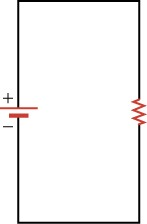1. If the current were not constant, the mobile charges would bunch up in places, which means that the voltage would decrease at that point. A lower voltage at some point would push the current in the direction that further decreases the voltage.
2. If the current were not constant, the mobile charges would bunch up in places, which means that the voltage would increase at that point. But a higher voltage at some point would push the current in the direction that decreases the voltage.
3. If the current were not constant, the mobile charges would bunch up in places, which mean that the voltage would increase at that point. A higher voltage at some point would push the current in the direction that further increases the voltage.
4. If the current were not constant, the mobile charges would bunch up in places, which mean that the voltage would decrease at that point. But a lower voltage at some point would push the current in the direction that increases the voltage.
35.

What is the current through each resistor in the circuit?

Figure 19.33
1. Current through resistors R1, R2, R3, and R4 is 0.48 A, 0.30 A, 1.2 A, and 0.24 A, respectively.
2. Current through resistors R1, R2, R3, and R4 is 1200 A, 1920 A, 480 A, and 2400 A, respectively.
3. Current through resistors R1, R2, R3, and is R4 2.08 A, 3.34 A, 0.833 A, and 4.17 A, respectively.
4. The same amount of current, 0.096 A, flows through all of the resistors.

#### 19.3Parallel Circuits

36.
In a house, a single incoming wire at a high potential with respect to the ground provides electric power. How are the appliances connected between this wire and the ground, in parallel or in series? Explain.
1. The appliances are connected in parallel to provide different voltage differences across each appliance.
2. The appliances are connected in parallel to provide the same voltage difference across each appliance.
3. The appliances are connected in series to provide the same voltage difference across each appliance.
4. The appliances are connected in series to provide different voltage differences across each appliance.

#### 19.4Electric Power

37.
A single resistor is connected across the terminals of a battery When you attach a second resistor in parallel with the first, does the power dissipated by the system change?
1. No, the power dissipated remain same.
2. Yes, the power dissipated increases.
3. Yes, the power dissipated decreases.
38.
In a flashlight, the batteries are normally connected in series. Why are they not connected in parallel?
1. Batteries are connected in series for higher voltage and power output.
2. Batteries are connected in series for lower voltage and power output.
3. Batteries are connected in series so that power output is a much lower for the same amount of voltage.
4. Batteries are connected in series to reduce the overall loss of energy from the circuit.